# User Reference:SpectralEstimator

## Function

The SpectralEstimator computes a continually updated estimate of the spectrum of its input data, i.e. the distribution of amplitude or energy over frequencies. Spectral estimation is done separately for each of the filter's input channels.

For each block of data, it uses a past window of data. Optionally, detrending is applied to the data in this window. Also, multiplication with a side lobe suppression window function may be performed, which is routinely done when estimating a spectrum from windowed data.

You may choose from two independent, and very different, spectral estimation algorithms, one based on Autoregression (AR), and one based on the Fast Fourier Transform (FFT).

### AR Algorithm

The AR algorithm computes an autoregressive model of its input data using the Maximum Entropy Method (Burg method). Its output may be raw AR coefficients, or an estimated power spectrum collected into bins.

AR coefficients are actually the coefficients of an all-pole linear filter that is fitted to reproduce the data's spectrum when applied to white noise.

Thus, the estimated power spectrum directly corresponds to that filter's transfer function, divided by the signal's total power. To obtain spectral power for finite-sized frequency bins, that power spectrum needs to be multiplied by total signal power, and integrated over the frequency ranges corresponding to individual bins.

This is done by numerical integration, evaluating the spectrum at equally spaced evaluation points, summing, and multiplying by bin width to obtain the power corresponding to a certain bin. For amplitude rather than power spectrum output, bin integrals are replaced with their square roots.

### FFT Algorithm

The FFT algorithm computes the Discrete Fourier Transform of its input data, i.e. it transforms its input into a representation in the frequency domain rather than the time domain. This is a linear transform, which may be viewed as expanding the input data in a new set of basis vectors, which correspond to equally spaced negative and positive frequency bins. The expansion coefficient (or Fourier coefficient) corresponding to a frequency component is a complex number, whose magnitude may be interpreted to represent signal amplitude in the associated frequency bin, and whose phase corresponds to the phase of the signal in that frequency bin.

In contrast to a naive DFT implementation, which requires time proportional to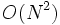$O(N^2)$, the FFT algorithm performs the computation in time proportional to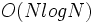$O(N logN)$ (with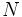$N$ being the number of input samples, which must be powers of two in the original FFT algorithm). The SpectralEstimation filter uses a generalization of the FFT algorithm, called FFTW, that can efficiently handle any number of input samples.

### Physical unit of the estimated spectrum

A power spectrum is an energy density distribution over frequencies. Thus, its physical dimension is "energy per frequency". The ARFilter actually computes a time series of such spectra, representing a flow of energy through each single point in the frequency domain. The proper physical dimension of such a flow is "energy per frequency per time".

In BCI2000, signal amplitude is measured in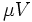$\mu V$, so signal energy is measured in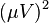$(\mu V)^2$. Measuring frequency in Hz, and time in seconds, these will cancel out in the physical unit's denominator. Thus, the physical unit of a power spectrum is$(\mu V)^2$, and$\mu V$ for an amplitude spectrum.

### Normalization of the estimated spectrum

Normalization is applied to energy, and chosen such that input energy per second matches output energy, summed over all bins. For an input signal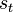$s_t$ and an output spectrum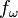$f_\omega$, this means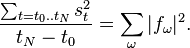$\frac{\sum_{t = t_0..t_N}s_t^2}{t_N - t_0} = \sum_{\omega}|f_\omega|^2.$

Normalization is applied for both AR and FFT algorithms in this way.

## Parameters

For all frequency-valued parameters, raw float values are interpreted in terms of the sampling rate; absolute frequencies may be given when followed with Hz, as in the following examples:

• 0.5 will always refer to the Nyquist frequency (half the sampling rate).
• 10.3Hz specifies a value of 10.3 Hz, regardless of the sampling rate.
• 15/2Hz specifies a value of 7.5 Hz.
• The unit must immediately follow the number.

### WindowLength

The length of the input data window over which the model/spectrum is computed, given as a time value in seconds (then immediately followed by the unit), or the number of signal blocks as in the following examples:

1.34s
500ms
5


### Detrend

Determines whether data are detrended prior to spectral estimation. Possible values are

• 0: no detrending,
• 1: mean removal,
• 2: linear trend removal.

### ModelOrder

The order of the autoregressive model. Roughly, this corresponds to the maximum number of peaks in the resulting spectrum. When using the FFT-based algorithm, this parameter is not used.

### OutputType

Possible values are

• 0: spectral amplitude,
• 1: spectral power,
• 2: AR coefficients.

If the output is a spectrum, the output signal's elements correspond to uniformly spaced frequency bins. For raw AR coefficients, the first output signal element contains total signal power, followed by the model's denominator coefficients.

### FirstBinCenter

A float value representing the center of the first frequency bin, e.g. "5Hz".

### LastBinCenter

A float value representing the center of the last frequency bin.

### BinWidth

A single nonnegative float value representing the width of a single bin, e.g. "3Hz". For the AR algorithm, this is the distance between points where the spectrum is evaluated. For the FFT algorithm, this determines the number of points over which the FFT is calculated (FFT size). If the WindowSize parameter is less than FFT size, the FFT's input is padded with zeros. If WindowSize is greater than FFT size, multiple FFTs are computed, and their results summed up, until all input data has been used.

### EvaluationsPerBin

A single nonnegative integer value representing the number of uniformly spaced evaluation points that enter into a single bin's value.

None.

## Examples

For a typical EEG application, you might use the following configuration:

ModelOrder= 16
FirstBinCenter= 0Hz
LastBinCenter= 30Hz
BinWidth= 3Hz
EvaluationsPerBin= 15


This will result in 11 bins, with the first bin covering the DC range from -1.5 to 1.5 Hz, which, due to symmetry of the transfer function, is twice the integral from 0 to 1.5 Hz. The last bin will cover the range from 28.5 Hz to 31.5 Hz. Evaluation points will be 0.2 Hz apart.